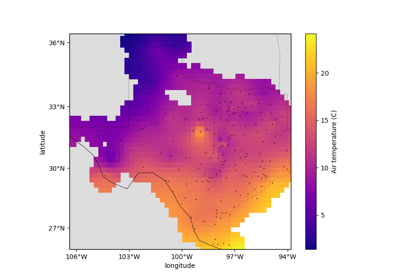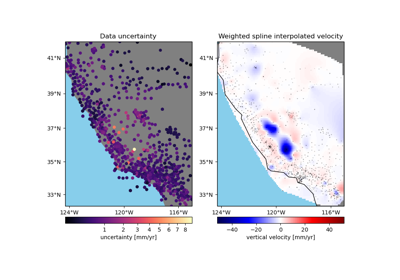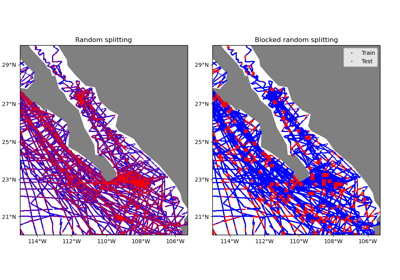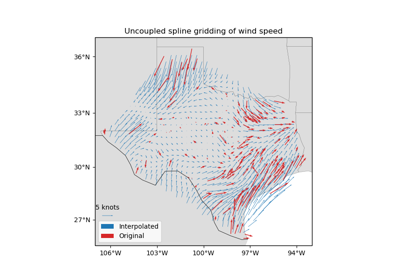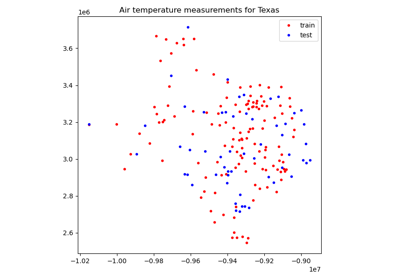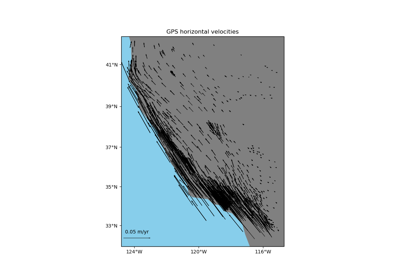# verde.train_test_split¶

verde.train_test_split(coordinates, data, weights=None, spacing=None, shape=None, **kwargs)[source]

Split a dataset into a training and a testing set for cross-validation.

Similar to sklearn.model_selection.train_test_split but is tuned to work on single- or multi-component spatial data with optional weights.

If arguments shape or spacing are provided, will group the data by spatial blocks before random splitting (using verde.BlockShuffleSplit instead of sklearn.model_selection.ShuffleSplit). The argument spacing specifies the size of the spatial blocks. Alternatively, use shape to specify the number of blocks in each dimension.

Extra keyword arguments will be passed to the cross-validation class. The exception is n_splits which is always 1.

Grouping by spatial blocks is preferred over plain random splits for spatial data to avoid overestimating validation scores. This can happen because of the inherent autocorrelation that is usually associated with this type of data (points that are close together are more likely to have similar values). See [Roberts_etal2017] for an overview of this topic. To use spatial blocking, you must provide a spacing or shape argument (see below).

Parameters
• coordinates (tuple of arrays) – Arrays with the coordinates of each data point. Should be in the following order: (easting, northing, vertical, …).

• data (array or tuple of arrays) – the data values of each data point. If the data has more than one component, data must be a tuple of arrays (one for each component).

• weights (none or array or tuple of arrays) – if not none, then the weights assigned to each data point. If more than one data component is provided, you must provide a weights array for each data component (if not none).

• spacing (float, tuple = (s_north, s_east), or None) – The spatial block size in the South-North and West-East directions, respectively. A single value means that the spacing is equal in both directions. If None, then shape must be provided in order to use spatial blocking.

• shape (tuple = (n_north, n_east) or None) – The number of blocks in the South-North and West-East directions, respectively. If None, then spacing must be provided in order to use spatial blocking.

Returns

train, test (tuples) – Each is a tuple = (coordinates, data, weights) generated by separating the input values randomly.

cross_val_score

Score an estimator/gridder using cross-validation.

BlockShuffleSplit

Random permutation of spatial blocks cross-validator.

Examples

To randomly split the data between training and testing sets:

>>> import numpy as np
>>> # Make some data
>>> data = np.array([10, 30, 50, 70])
>>> coordinates = (np.arange(4), np.arange(-4, 0))
>>> train, test = train_test_split(coordinates, data, random_state=0)
>>> # The training set:
>>> print("coords:", train)
coords: (array([3, 1, 0]), array([-1, -3, -4]))
>>> print("data:", train)
data: (array([70, 30, 10]),)
>>> # The testing set:
>>> print("coords:", test)
coords: (array(), array([-2]))
>>> print("data:", test)
data: (array(),)


If weights are given, they will also be split among the sets:

>>> weights = np.array([4, 3, 2, 5])
>>> train, test = train_test_split(
...     coordinates, data, weights, random_state=0,
... )
>>> # The training set:
>>> print("coords:", train)
coords: (array([3, 1, 0]), array([-1, -3, -4]))
>>> print("data:", train)
data: (array([70, 30, 10]),)
>>> print("weights:", train)
weights: (array([5, 3, 4]),)
>>> # The testing set:
>>> print("coords:", test)
coords: (array(), array([-2]))
>>> print("data:", test)
data: (array(),)
>>> print("weights:", test)
weights: (array(),)


Data with multiple components can also be split:

>>> data = (np.array([10, 30, 50, 70]), np.array([-70, -50, -30, -10]))
>>> train, test = train_test_split(coordinates, data, random_state=0)
>>> # The training set:
>>> print("coords:", train)
coords: (array([3, 1, 0]), array([-1, -3, -4]))
>>> print("data:", train)
data: (array([70, 30, 10]), array([-10, -50, -70]))
>>> # The testing set:
>>> print("coords:", test)
coords: (array(), array([-2]))
>>> print("data:", test)
data: (array(), array([-30]))


To split data grouped in spatial blocks:

>>> from verde import grid_coordinates
>>> # Make a regular grid of data points
>>> coordinates = grid_coordinates(region=(0, 3, 4, 7), spacing=1)
>>> data = np.arange(16).reshape((4, 4))
>>> # We must specify the size of the blocks via the spacing argument.
>>> # Blocks of 1.5 will split the domain into 4 blocks.
>>> train, test = train_test_split(
...     coordinates, data, random_state=0, spacing=1.5,
... )
>>> # The training set:
>>> print("coords:", train, train, sep="\n")
coords:
[0. 1. 2. 3. 0. 1. 2. 3. 2. 3. 2. 3.]
[4. 4. 4. 4. 5. 5. 5. 5. 6. 6. 7. 7.]
>>> print("data:", train)
data: (array([ 0,  1,  2,  3,  4,  5,  6,  7, 10, 11, 14, 15]),)
>>> # The testing set:
>>> print("coords:", test, test)
coords: [0. 1. 0. 1.] [6. 6. 7. 7.]
>>> print("data:", test)
data: (array([ 8,  9, 12, 13]),)


## Examples using verde.train_test_split¶